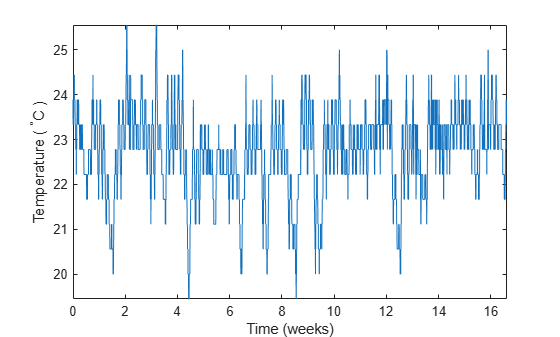# 使用频率分析求周期性

```load officetemp tempC = (temp - 32)*5/9; fs = 2*24*7; t = (0:length(tempC) - 1)/fs; plot(t,tempC) xlabel('Time (weeks)') ylabel('Temperature ( {}^\circC )') axis tight``````tempnorm = tempC - mean(tempC); [pxx,f] = periodogram(tempnorm,[],[],fs); plot(f,pxx) ax = gca; ax.XLim = [0 10]; xlabel('Frequency (cycles/week)') ylabel('Magnitude')```